Online Tution   »   Education News   »   Class 12 Math Board Paper 2023

# Class 12 Math Board Paper 2023 [ Expected for 11 March ]

## Class 12 Math Board Paper Expected

Expected Class 12 Math Board Paper 2023: The CBSE Class 12 Mathematics Examination will be held on March 11, 2023. Arithmetic requires practise. Therefore now is a critical time to optimise our Class 12 Mathematics Exam result. Students should practise the Sample Class 12 Mathematics Board Paper 2023 as much as they can in order to be prepared to answer questions in the exam hall.

Our Adda247 Expert faculty prepares the Expected Class 12 Math Board Paper 2023 for the benefit of class 12 candidates, which is based on the genuine Cbse Class 12 Exam pattern. Applicants who plan to sit the Class 12 Math Board Paper 2023 must do so.## Class 12 Math Board Paper 2023 Most Expected Questions

Class 12 Maths Important Question 2023

Class 12 Maths Deleted Syllabus 2023

Class 12 Maths Practice Paper 2023

Class 12 Maths Syllabus 2023

Class 12 Maths Answer key 2023## Class 12 Maths Board Paper 2023 Revision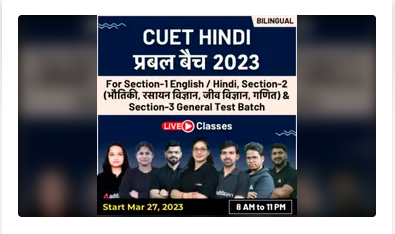## Maths Class 12 Board Expected Question Paper

Adda247’s Class 12 Board Mathematics Board Paper 2023, like the official CBSE Class 12 Maths sample paper, comprises five sections for an overall score of 70. Check out the below mark distribution for the Class 12 Maths Board Paper 2023.

• This Question paper contains – five sections A, B, C, D and E. Each section is compulsory. However, there are internal choices in some questions.
• Section A has 18 MCQ’s and 02 Assertion-Reason based questions of 1 mark each.
•  Section B has 5 Very Short Answer (VSA)-type questions of 2 marks each.
• Section C has 6 Short Answer (SA)-type questions of 3 marks each.
• Section D has 4 Long Answer (LA)-type questions of 5 marks each.
• Section E has 3 source based/case based/passage based/integrated units of assessment (4 marks each) with sub parts.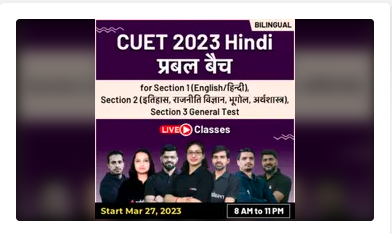## Class 12 Maths Board Paper 2023-Section A

SECTION A

Q1. Let A = { 1 , 2, 3 } and consider the relation R = {(1 , 1), (2 , 2), ( 3 , 3), (1 , 2), (2 , 3), (1,3)} Then , R is

(a) Reflexive but not symmetric
(b) Reflexive but not transitive
(c) Symmetric and transitive
(d)Neither symmetric nor transitive
Q2. If A is a skew – symmetric matrix , then A² is

(a) Symmetric
(b) Skew – symmetric
(c) A² = A
(d) A² ≠ A

Q3. The number of arbitrary constants in the general solution of a differential equation of fourth order are :
(a) 0
(b) 2
(c) 3
(d) 4

Q4. If y = log x/ (1+x) , then dy/ dx is equal to

(a) x/ (1+x)²
(b) 1/ (1+x) ²
(c) 2x/ (1+x)²
(d) 1/ x(1+x)

Q5. Corner points of the region for an LPP are (0, 2), (3, 0), (6, 0),(6, 8), and (0, 5) . Let F = 4x + 6y be the objective function. The minimum value of F occurs at
(a) (0,2) only
(b)(3,0) only
(c) The midpoint of the line segment joining the points (0,2) and ( 3, 0) only
(d) Any point on the line segment joining the points (0,2) and (3, 0) only.

Q6. f(x) = xˣ has a stationary point at
(a) x = e
(b) x = 1/ e
(c) x = 1
(d) x = √eQ7. If A and B are events such that P ( A/ B) = P (B/ A) , then ………

(a) P(A) = P(B)
(b) P(A) ≠ P(B)
c) P(A) + P(B) = 1
(d None of these

Q8. If R = {(x , y ): x + 2y = 8 } is a relation N , then the range of R is :
(a){3, 2 , 1}
(b) { 3 , 2}
(c) {2 , 8 , 1}
(d) {3}

Q9. Let f ∶ R → R be defined as f(x) = 3x. Choose the correct answer.

(a) f is one – one onto
(b) f is many-one onto
(c) f is one – one but not onto
(d) f is neither one-one nor onto

Q10. The principal value of [tan-1 √3 − cot-1, (−√3)] is :

(a)π
(b) − π/2
(c) 0
(d) 2√3
Q11. If cos (sin-1 2/ 5 + cos-1 x) = 0 , then x is equal to

(a) 1/ 5
(b)2/ 5
(c) 0
(d) 1
Q12. If a = 3î− 2ĵ+ k̂ and b= 2î+ ĵ, then value of a .  b is equal to …………
(a) 2
(b) 3
(c) 4
(d) 0
Q13. The value of ∫ (cos 2x)/ (sinx +cosx)² dx is
(a) log | cos x + sin x | + C
(b) log | cos x − sin x | + C
(c) log | cos x + sin x |² + C
(d) log | cos x + sin x |-2 + C
Q14. The derivative of 3ˣ  w.r.t x is :

(a) log 3
(b) x. 3ˣ-1
(c) 0
(d) 3ˣ log 3

Q15. A is a square matrix of order 2, then adj(adj A) is :
(a) O
(b) I
(c) A-1
(d) A

Q16. The number of arbitrary constants in the general solution of a differential equation of fourth order is :
(a) 1
(b) 2
(c) 3
(d) 4

Q17. If A and B are independent , then P(exactly one of A , B occurs ) = P(A)P′ (B) + P(B)P′(A)

(a)True
(b) False
(c) Can’t say
(d) data insufficient

Q18. f Cij denotes the cofactor of element Pij of the matrix P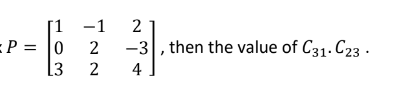(a) 5
(b) 24

(c)-24
(d) -5
Direction (Q19 – Q20 ) : In the following questions , a statement of assertion (A) is followed by a statement of Reason (R) .Choose the correct answer out of the following choices.
(a)Both A and R are true and R is the correct explanation of A.
(b)Both A and R are true and R is not the correct explanation of A.
(c)A is true but R is false.
(d)A is false but R is true.Q19 Assertion(A) : The vector equation of the line through the points (3 , 4 , -7) and ( 1 , -1 , 6) is (x − 3)î+ (y − 4)ĵ+ (z + k)k̂ = λ(−2î− 5ĵ+ 13k̂)
Reason (R): The vector equation of a line passes through two vectors a and b is given
by r = a + λ(b − a ) .

Q20. Assertion (A) : If P(A) = 6/11, P(B) = 5/ 11 and P(A ⋃ B) = 7/11 then the value of P(A|B) is 4 / 5

Reason (R) : P(A |B) = P(A ⋂ B)/ P(B) where P(A ⋂ B) = P(A) + P(B) − P(A ⋃ B)## Class 12 Maths Board Exam Paper 2023 Section B

SECTION B

Q21.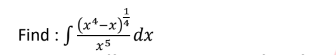Q22. Form the differential equation of the family of circles having a centre on y-axis and radius 3 units.

Or

Find the sum of the order and the degree of the following differential equation :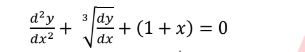Q23. If a = î+ 2ĵ+ k̂ , b= î− ĵ then find the value of | a × b |
Or
If a , b are two vectors such that |a + b | = |a | , then prove that 2a + b⃗, is perpendicular to b .

Q.24.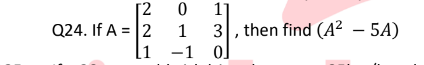Q25. If a 20 years old girl drives her car at 25km/hr , she has to spend Rs 4/km on petrol. If she drives her car at 40 km/h , the petrol cost increases to Rs 5/km .She has Rs . 200 to spend on petrol and wishes to find the maximum distance she can travel within one hour .Express the above problem as a linear programming problem.

Get Class 12th Boards Ka Vardaan eBook Free Use Code CLASS12## Class 12 Maths Board Expected Paper 2023 Section C

SECTION C

Q26. The members of a consulting firm rent cars from three rental agencies :
50% from agency X , 30% from agency Y and 20% from agency Z. From past experience ,it is known that 9% of the cars from agency X need a service and tuning before renting , 12% of the cars from agency Y need a service and tuning before renting and 10% of the cars from agency Z need a service and tuning before renting. If the rental car delivered to the firm needs service and tuning , find the probability that agency Z is not to be blamed.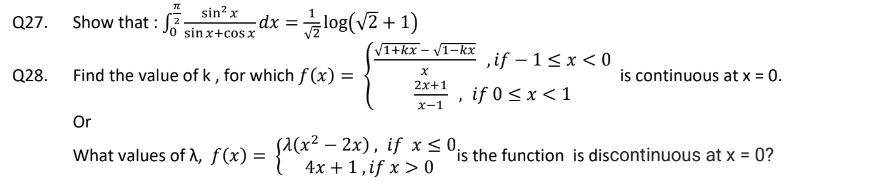Q29. Find the equations of the planes that passes through three points (1, 1, 0), (1, 2, 1), and (– 2, 2, – 1).

Or

Find the shortest distance between the lines r= (4î− ĵ) + λ(î+ 2ĵ− 3k̂) and r= (î− ĵ + 2k̂) + μ(2î+ 4ĵ− 5k̂)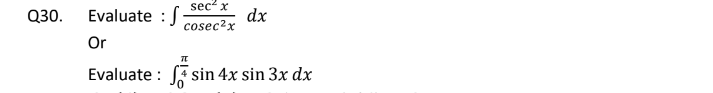Q31. If P(A) = 0.8 , P(B) = 0.5 and P(B|A) = 0.4 .Find
(i)P(A ⋂ B)
(ii)P(A|B)
(iii)P(A ⋃ B)## Class 12 Maths Board Exam Paper 2023-Section D

SECTION D

Q32.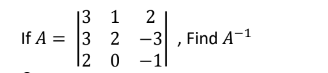Or

Solve the linear system of equation :
x + 3y – 2z = 0
2x – 3y + z = 1
4x – 3y + z = 3

Q33. Find the area bounded by the curves y = √x , 2y + 3 = x and x −axis

Or

Find the area of the region : {(x , y ): x² + y² ≤ 8 , x² ≤ 2y}

Q34. Solve the following linear programming problem graphically:
Minimize Z = 200 x + 500 y
subject to the constraints:
x + 2y ≥ 10
3x + 4y ≤ 24
x ≥ 0, y ≥ 0

Q35. In a hockey match, both teams A and B scored same number of goals up to the end of the game, so to decide the winner, the referee asked both the captains to throw a die alternately and decided that the team, whose captain gets a six first, will be declared the winner. If the captain of team A was asked to start, find their respective probabilities of winning the match and state whether the decision of the referee was fair or not.

## Expected Class 12 Physics Board Exam Paper 2023- Section E

SECTION E

Q36. To teach the application of probability a maths teacher arranged a surprise game for 5 of his students namely Archit, Aadya, Mivaan, Deepak and Vrinda. He took a bowl containing tickets numbered 1 to 50 and told the students go one by one and draw two tickets simultaneously from the bowl and replace it after noting the numbers.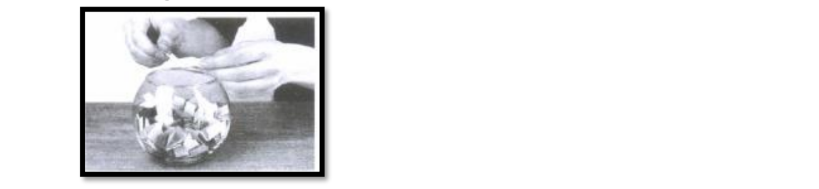(i) Teacher ask Vrinda, what is the probability that both tickets drawn by Archit shows even number?
(ii) Teacher ask Mivaan, what is the probability that both tickets drawn by Aadya shows odd number?
(iii) Teacher ask Deepak, what is the probability that tickets drawn by Mivaan, shows a multiple of 4 on one ticket and a multiple 5 on other ticket?

Q37. A mobile tower stands at the top of a hill. Consider the surface on which tower stand as a plane having points A(0, 1,2), B(3, 4, -1), and C(2, 4, 2) on it. The mobile tower is tied with 3 cables from point A, Band C such that it stands vertically on the ground. The peak of the tower is at the point (6, 5, 9), as shown in the figure.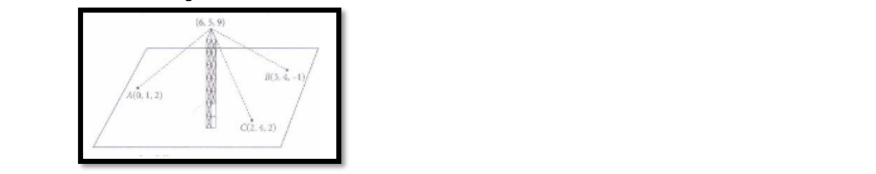Based on the above information, answer the following questions
(i) The equation of plane passing through the points A, Band C is
(ii) The height of the tower from the ground is
(iii) The equation of line of perpendicular drawn from the peak of tower to the ground is.

Q38. The given Integral ∫ f(x)dx can be transformed into another form by changing the independent variable x to t by substituting x = g(t)

Consider I = ∫ f(x)dx
Put x = g(t) so that dx/ dt = g′(t)

we write dx = g′(t)dt

Thus I = ∫ f(x)dx = ∫ f(g(t))g′ (t)dt

This change of variable formula is one of the important tools available to us in the name of integration by substitution.

## Class 12 Board Maths Exam Paper- With Solutions

We also provide full solutions for the Class 12 Mathematics Board Exam Paper 2023. Students can examine their grades after completing the Anticipated Class 12 mathematics board paper 2023.

Sharing is caring!

## FAQs

### When will CBSE class 12 Math Exam takes place?

The CBSE Class 12 Maths Examination is going to take place on 5th March 2023.

### How many Sections are there in the Class 12 Board Math Exam Paper 2023?

The Class 12 Board Physics Exam Paper 2023 comprises five sections for an overall score of 80.

### What is the totals marks of Class 12 Board Math Exam Paper 2023?

The full mark of the Class 12 Board Math Question Paper is 70 Marks.

### Is Class 12 Math hard?

Many CBSE Class 12 math students who took the CBSE 12th Mathematics test 2023 in the previous year reported that the paper's difficulty level was moderate and that it was a little lengthy.

### Which is the hardest topic in maths 12th?

The hardest chapters in class 12 math are Chapter 8 Applications of Integrals, Chapter 11 Three Dimensional Geometry, and Chapter 13 Probability. They take time to understand and are difficult.

### How many Chapters are there in the Class 12 Maths Syllabus 2023?

The class 12 math curriculum consists of a total of 13 chapters.

### TOPICS:

[related_posts_view]